cancel
Showing results for
Search instead for
Did you mean: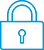It has been a while since anyone has replied. Simply ask a new question if you would like to start the discussion again.Level 4
138 92 2 5
Message 1 of 9
1,485
Flag Post

Solved!

# How to write a square root elements in diagonal of square matrixHP Recommended
HP 50 g

Hello HP community ,

I have one question , I' m going little bit programming in User RPL and i have one problem that I didn't solve jet.

Here is example of my task.

I have square matrix of any dimension (nxn) and I want to write a vector ( n x 1 )  which have elements equal to a square root of individually elements in square matrix at its main diagonal  , and of course i want to store the vector (nx1) into global variable .

How to write this type of program , please help me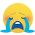Can anyone write me an answer , i have code for this program but is not good because my program prints a numbers without a sign of vector when i store these number to array .

I have also a simbollicaly a picture , what I supposed to do with this program .

there is my source code ...

<< 3 SF Qxx SIZE 1 GET 'DIM' STO 1 DIM

FOR  i 1 DIM

FOR j

IF i j ==

THEN Qxx { i j }

GET square root sign

END

NEXT

NEXT

>>

Please Help me HP community i don't know what to do .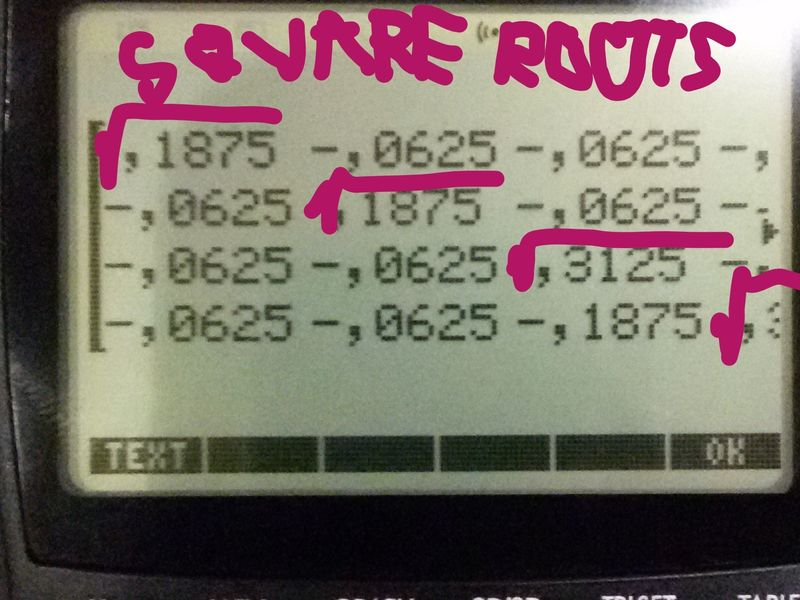square roots of elements in main diagonal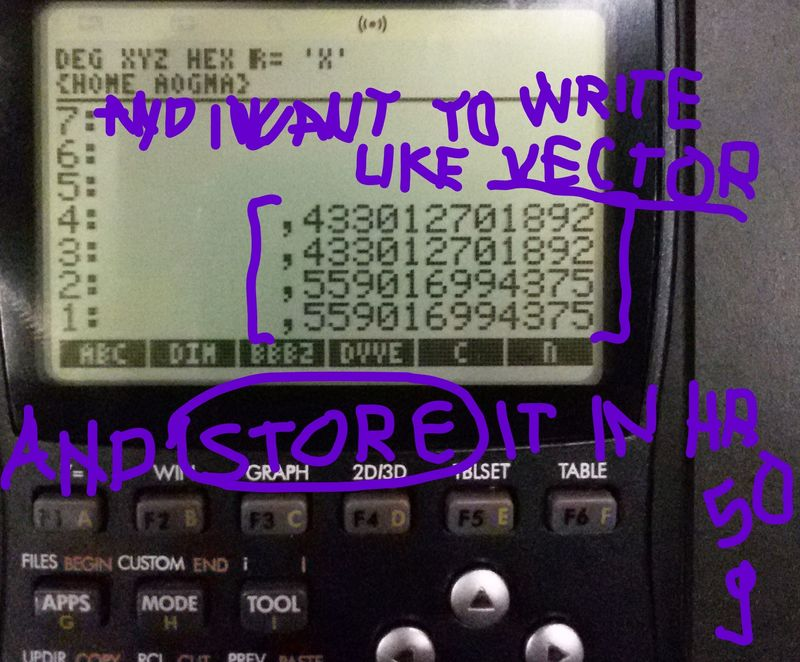... and vector with squareroots of elements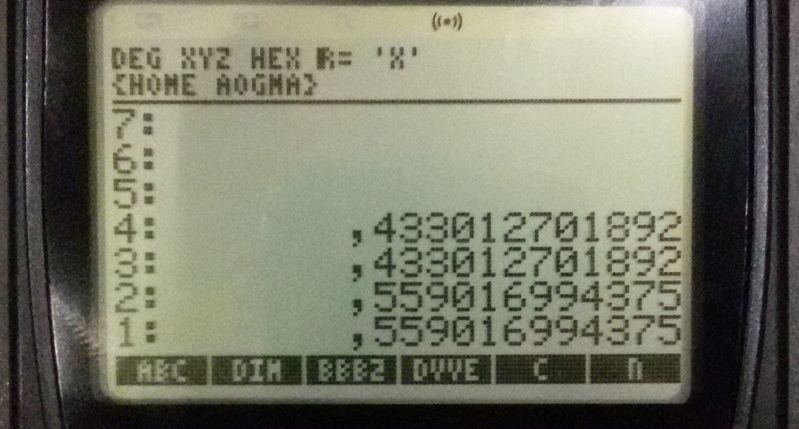i get something like that...

Tags (1)
8 REPLIES 8Level 8
624 611 118 208
Message 2 of 9
Flag PostHP Recommended

Not sure I understand what you're seeking, but if you want to extract the diagonal of a square matrix into a vector, and square root its elements individually, here's one way to do that (replace "sqrt" with the square root symbol):

→DIAG  AXL  sqrt  AXL

Here's another way:

→DIAG  «  sqrt  »  MAP

Disclaimer: I don't work for HP. I'm just another happy HP calculator user.

-Joe-Level 4
138 92 2 5
Message 3 of 9
Flag PostHP Recommended

f yes thanks , but i have one task for these vector , i want also transponse that vector and multiplied them with the real number or one another vector , maybe better said matrix dim. n x 1 , when I going to do that it shows me something like that ( in the picture below )

And do you know easiest way to get a squareroots of elements in main diagonal in form of square matrix but i want that does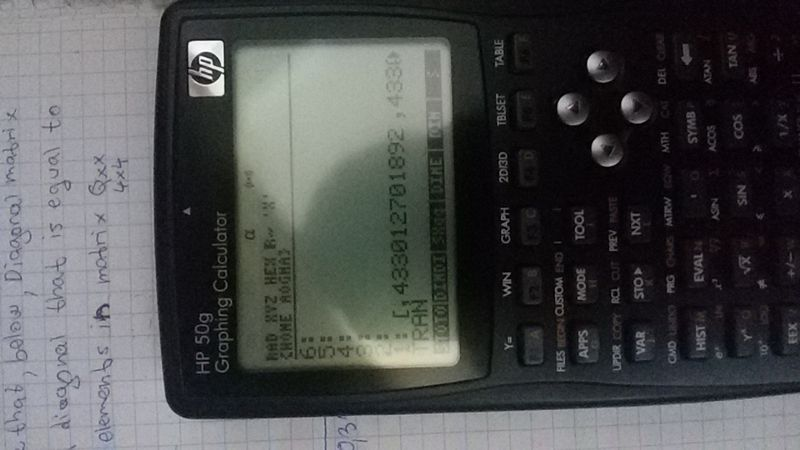When I m going to hit TRAN ...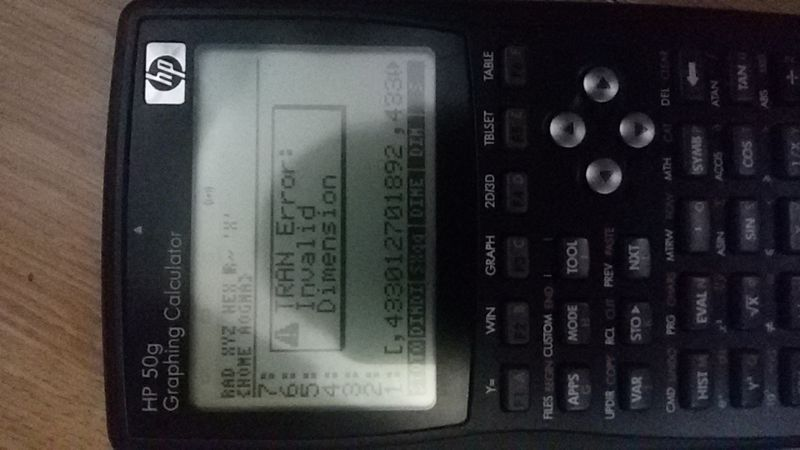It s shows me that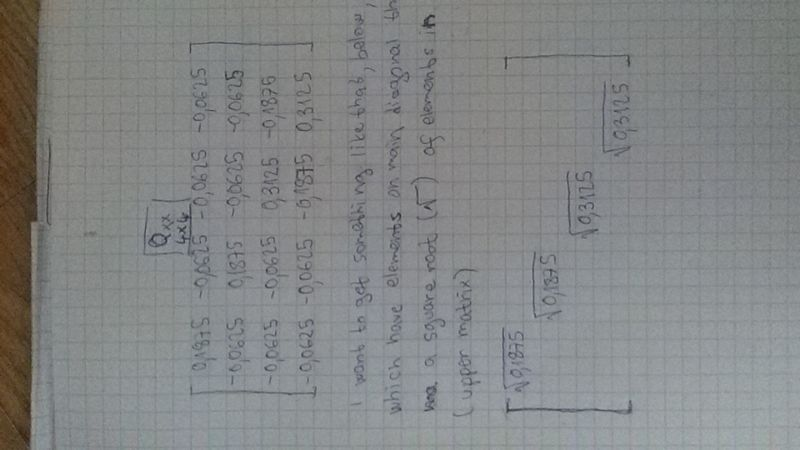Another task how to get diagonal matrix of squareroot elements in previous matrix...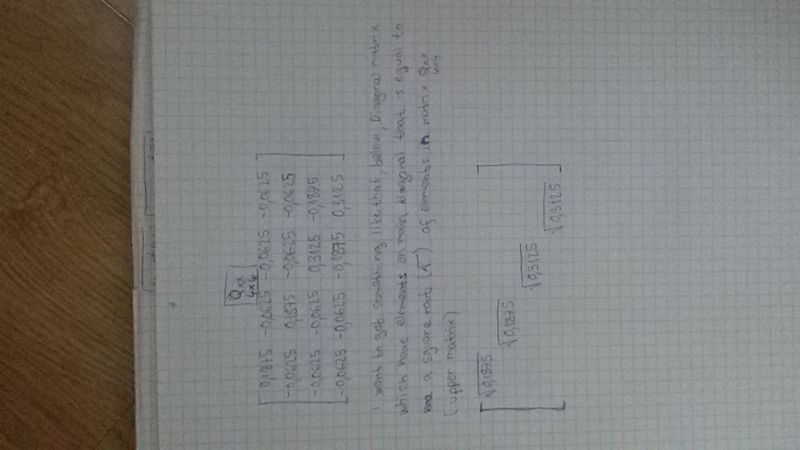Little explanation... works on any dimensions of matrix , of course i have picture for that also , i would be very very gretefull if you give me the answer on that question ,

But for now you've helped me a lot , thank you colleage 😃Level 6
305 297 32 76
Message 4 of 9
Flag PostHP Recommended

When I understand you well you have a vector of the form [2,4,7,2] and you want to transform it into a column matrix of the form [,,,].

I would do this: first transform your vector into a list with AXL (with thanks to Joe)
Then evaluate your list, so this puts the elements on the stack.
Then enter the list: {n,1}.
n is the number of rows and 1 is the number of columns
Then use →ARRY.
This transforms the elements on the stack to a matrix of size n*1, which is what you want.

EDIT

I understand that you already have the elements of the vector on the stack.

So the only thing left to do is entering {n,1} followed by →ARRYLevel 8
624 611 118 208
Message 5 of 9
Flag PostHP Recommended

The fastest way to turn a vector into a matrix is with the RDM (ReDiMension) command:

[7, 8, 9] {3 1} RDM --> [, , ] (a column matrix)

[7, 8, 9] {1 3} RDM --> [[7, 8, 9]] (a row matrix)

A way to programmatically turn any vector into a column matrix is DUP SIZE 1 + RDM.

-Joe-

-Joe-
Tags (1)Level 4
138 92 2 5
Message 6 of 9
Flag PostHP Recommended
I don't know what to say , thank you both , guys , you helped me a lot with this issue .Level 6
305 297 32 76
Message 7 of 9
Flag PostHP Recommended

I now realize that the method I suggested in message 4 was not very good. Beginner's work!

Of course Joe's method is much better.

Still another way to transform  [2,4,7,2] into [,,,] would be:

Use OBJ →    to put the elements of the vector on the stack.

Level 1 will now contain {n}  (n is the length of the vector)

Add 1, level 1 will now contain {n,1}

Use   →ARRY to transform the elements on the stack into a n*1 matrix.

Also, apart from the command GET, the command PUT is very important.

Because your aim was to construct a n*1 matrix you could have created such a  matrix with

CON ({n,1},0)

This command creates a matrix with dimensions n*1, where all elements are constants, in this case equal to 0.

Next store this matrix in variabel M1.

Next, when you would like element (3,1) of this matrix to be 7, just use:

PUT (M1,{3,1},7)Level 6
305 297 32 76
Message 8 of 9
Flag PostHP Recommended

You also wanted to know how to construct a matrix with same dimension as original matrix, with all elements equal to 0, except the diagonal elements, which are equal to the square root of the diagonal elements of the original matrix.

You know already how to obtain a vector with these elements.
You are a lucky guy because there is a command for this.
When the vector is on the stack you just have to write:
DIM DIAG→

The command is described in both the ordinary User's Guide and the Advanced one:

Both manuals are very useful, the second one mainly (at least for me) because it describes all commands.

You could also write a program which creates such a matrix by using a loop, and the commands CON, GET and PUT.

Such a program could be:

{DIM DIM} 0 CON
'M1' STO
1 DIM FOR i
'M1' {i i}
Qxx {i i} GET sqrt
PUT
NEXT
M1

Here Qxx is the name of your original matrix, with dimension DIM*DIM

Explanation:

{DIM DIM} 0 CON:
creates a square matrix with all elements equal to 0 and dimension DIM*DIM. (CON=constant)

'M1' STO: stores this matrix in variable M1.

1 DIM FOR i : starts the loop.

Qxx {i i} GET sqrt : takes the roots of the diagonal elements of Qxx.

'M1' {i i} ....PUT: puts them into M1Level 4
138 92 2 5
Message 9 of 9
Flag PostHP Recommended
Oooh , yes great solutions from You mr. Jan_D , I don't know which is better , thank you very much , I'm so sorry because I replied so late , I were so bussy , ok , Thank you once again !!!😃😃😃
† The opinions expressed above are the personal opinions of the authors, not of HP. By using this site, you accept the Terms of Use and Rules of Participation